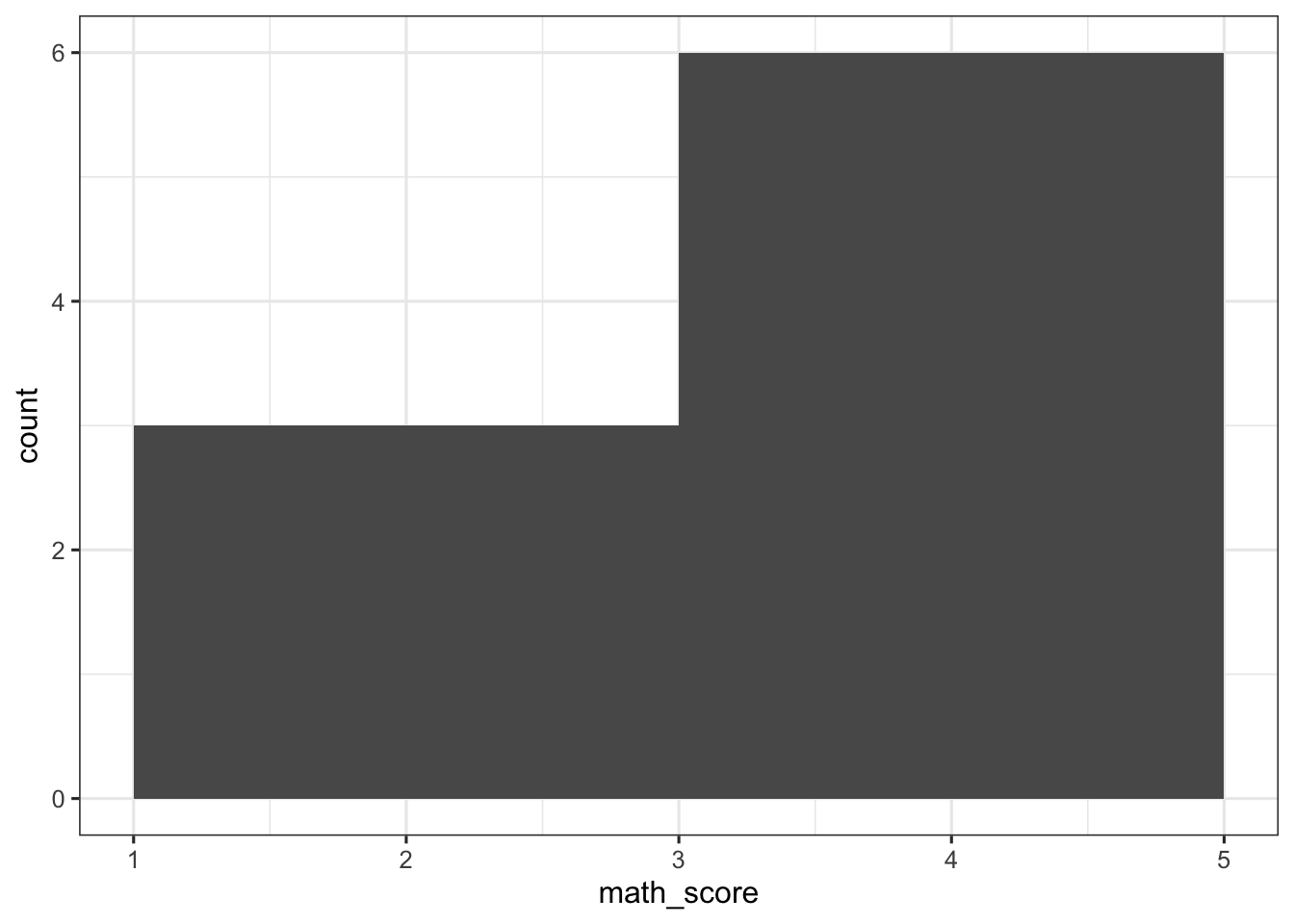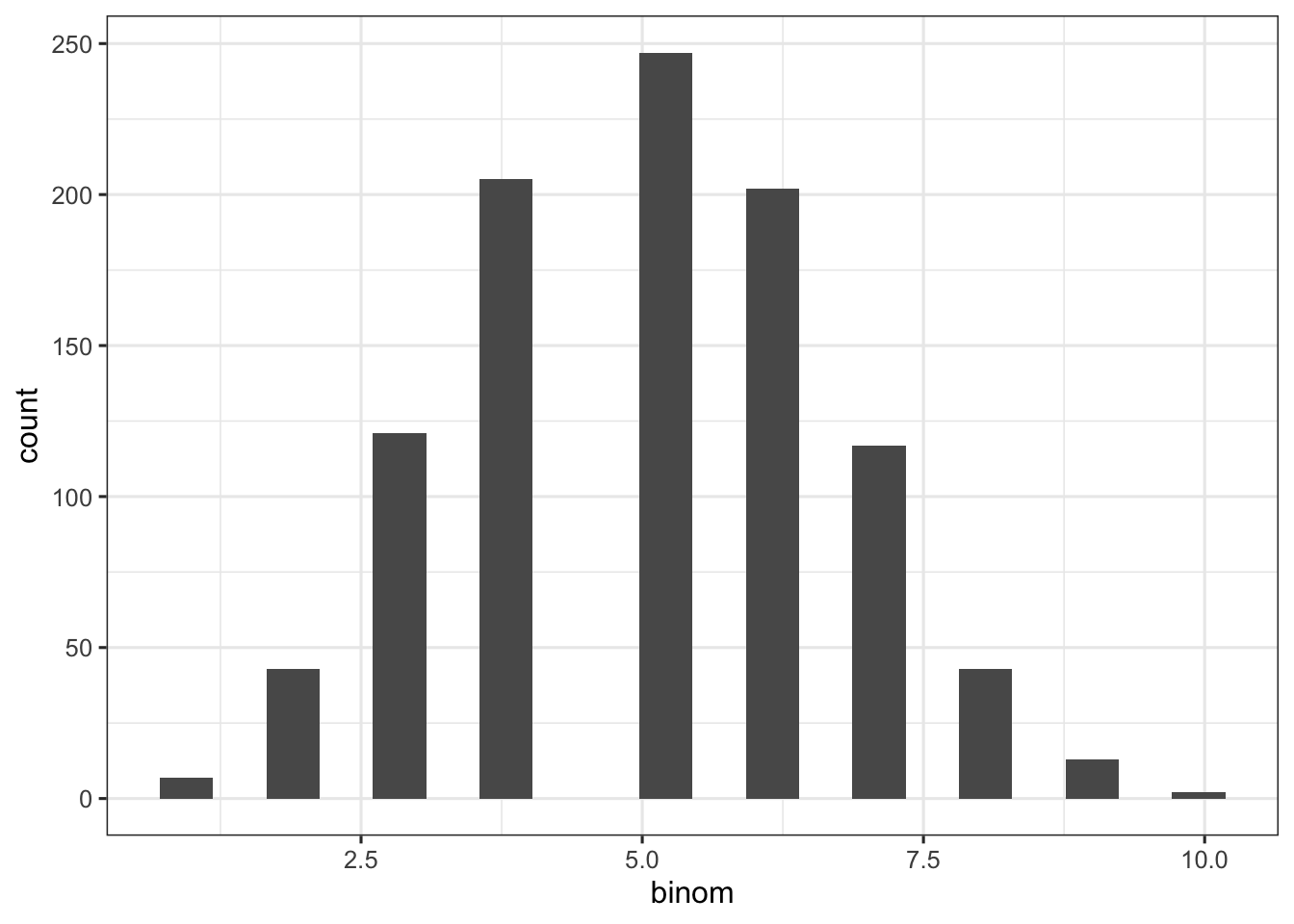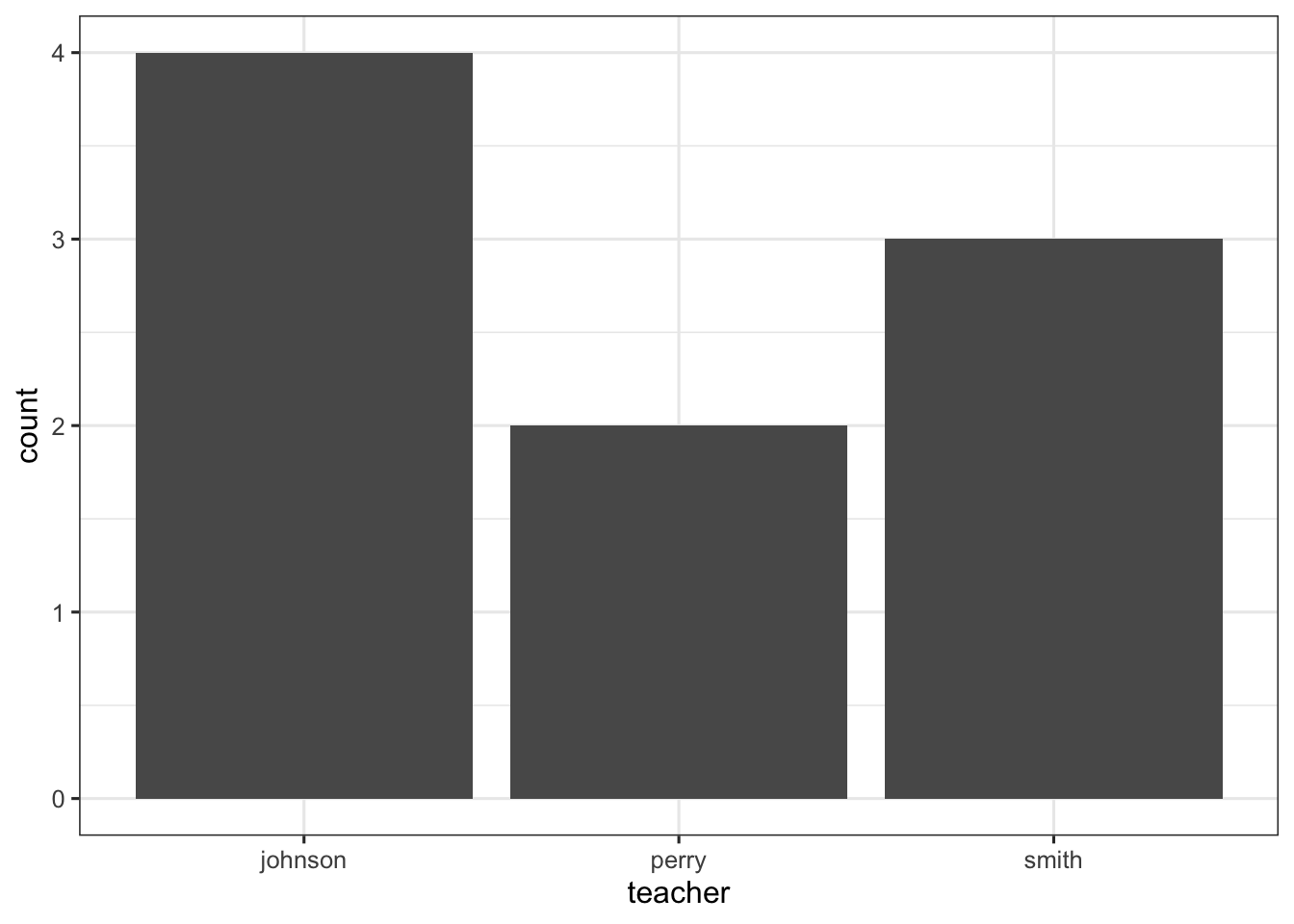# Getting started

``````library(tidyverse)
theme_set(theme_bw(base_size = 12))
set.seed(1)``````

This website — https://ggplot2.tidyverse.org/reference/ — is incredibly useful. We’ll focus on the most basic building blocks. Let’s build a couple of data frames to use:

``````scores <-
tibble(
name = c("mike", "carol", "greg", "marcia", "peter", "jan", "bobby", "cindy", "alice"),
school = c("south", "south", "south", "south", "north", "north", "north", "south", "south"),
teacher = c("johnson", "johnson", "johnson", "johnson",  "smith", "smith", "smith", "perry", "perry"),
math_score = c(4, 3, 2, 4, 3, 4, 5, 4, 5),
reading_score = c(1, 5, 2, 4, 5, 4, 1, 5, 4)
)

random_numbers <-
tibble(
binom = rbinom(1000, 10, 0.5),
pois = rpois(1000, 5)
) %>%
mutate(
mix = rnorm(1000, binom + pois, 5),
high_low = ifelse(mix > 10, "high", "low")
)``````

To get the basics down, I’ll show an example using the `scores` dataset and then ask you to make a similar plot using the `random_numbers` dataset.

# Plotting one variable

## geom_histogram

If your variable is continuous, you’ll want to use `geom_histogram`:

``````scores %>%
ggplot(aes(x = math_score)) +
geom_histogram(binwidth = 2)````````?geom_histogram``

Make a histogram with the random numbers and play around with `geom_histogram` options:

``````random_numbers %>%
ggplot(aes(x = binom)) +
geom_histogram(bins = 20)``````## geom_bar

If your variable is discrete, you’ll want to use `geom_bar`:

``````scores %>%
ggplot(aes(x = teacher)) +
geom_bar()``````Your turn with `geom_bar`:

``````random_numbers %>%
ggplot(aes(x = high_low)) +
geom_bar() +
coord_flip()``````